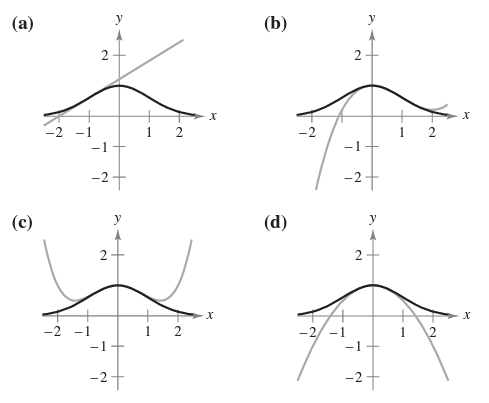×
Get Full Access to Calculus: Early Transcendental Functions - 6 Edition - Chapter 9.7 - Problem 3
Get Full Access to Calculus: Early Transcendental Functions - 6 Edition - Chapter 9.7 - Problem 3

×

# ?Matching In Exercises 1-4, match the Taylor polynomial approximation of the function $$f(x)=e^{-x^{2} / 2}$$ with the corresponding graph. [The graphsISBN: 9781285774770 141

## Solution for problem 3 Chapter 9.7

Calculus: Early Transcendental Functions | 6th Edition

• Textbook Solutions
• 2901 Step-by-step solutions solved by professors and subject experts
• Get 24/7 help from StudySoup virtual teaching assistantsCalculus: Early Transcendental Functions | 6th Edition

4 5 1 262 Reviews
14
2
Problem 3

Matching In Exercises 1-4, match the Taylor polynomial approximation of the function $$f(x)=e^{-x^{2} / 2}$$ with the corresponding graph. [The graphs are labeled (a), b), (c), and (d).]$$g(x)=e^{-1 / 2}[(x+1)+1]$$

Text Transcription:

g(x)=e^-1/2[(x+1)+1]

Step-by-Step Solution:

Step 1 of 5) Let’s take a closer look at the arctangent, arccotangent, arcsecant, and arccosecant functions.

Step 2 of 2

##### ISBN: 9781285774770

This full solution covers the following key subjects: . This expansive textbook survival guide covers 134 chapters, and 10738 solutions. Calculus: Early Transcendental Functions was written by and is associated to the ISBN: 9781285774770. The answer to “?Matching In Exercises 1-4, match the Taylor polynomial approximation of the function $$f(x)=e^{-x^{2} / 2}$$ with the corresponding graph. [The graphs are labeled (a), b), (c), and (d).] $$g(x)=e^{-1 / 2}[(x+1)+1]$$Text Transcription:g(x)=e^-1/2[(x+1)+1]” is broken down into a number of easy to follow steps, and 32 words. The full step-by-step solution to problem: 3 from chapter: 9.7 was answered by , our top Calculus solution expert on 11/14/17, 10:53PM. This textbook survival guide was created for the textbook: Calculus: Early Transcendental Functions, edition: 6. Since the solution to 3 from 9.7 chapter was answered, more than 268 students have viewed the full step-by-step answer.

## Discover and learn what students are asking

Calculus: Early Transcendental Functions : Inverse Trigonometric Functions: Integration
?In Exercises 1-20, find the indefinite integral. $$\int \frac{d x}{\sqrt{1-4 x^{2}}}$$

Calculus: Early Transcendental Functions : Change of Variables: Polar Coordinates
?Describing a Region In Exercises 5-8, use polar coordinates to describe the region shown.

Statistics: Informed Decisions Using Data : Scatter Diagrams and Correlation
?True or False: Correlation implies causation.

Statistics: Informed Decisions Using Data : Applications of the Normal Distribution
?Explain why P(X < 30) should be reported as < 60.0001 if X is a normal random variable with mean 100 and standard deviation 15.

Unlock Textbook Solution

?Matching In Exercises 1-4, match the Taylor polynomial approximation of the function $$f(x)=e^{-x^{2} / 2}$$ with the corresponding graph. [The graphs